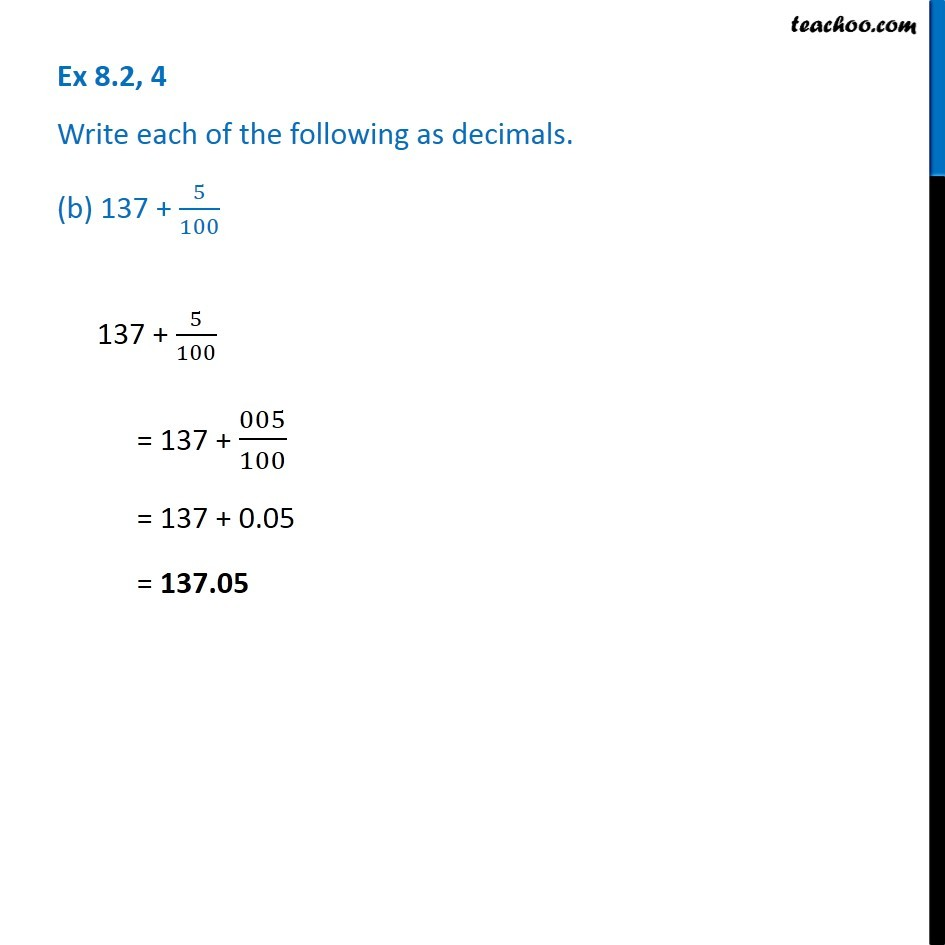Ex 8.2

Chapter 8 Class 6 Decimals
Serial order wiseGet live Maths 1-on-1 Classs - Class 6 to 12

### Transcript

Ex 8.2, 4 Write each of the following as decimals. (b) 137 + 5/100137 + 5/100 = 137 + 005/100 = 137 + 0.05 = 137.05